# Chepakovich Valuation Model

#### Summary

Unlike other models, the Chepakovich valuation model has almost universal applicability. For intrinsic value estimations it does not require the company to pay dividends or even have positive net income. Nor does it need detailed projections of future cash flows. All is needed is financial statements for the last several years and an estimation of future revenue growth rates. The model employs an original method for splitting total expenses into fixed and variable expenses from the income statement data. This, combined with projected revenue growth rates, makes possible forecasting of the company's future profitability. The model also has a number of other unique features not present in other valuation models: variable period-dependent discount rates; a direct link between the magnitude of revenues and that of production assets needed to sustain a given level of revenues; optimal and maximum levels of financial leverage that allow for an extraordinary divided to be paid out in case of underleverage or limit the revenue growth rate in case of overleverage; estimation of future actual stock-based compensation expenses (as opposed to the accounting ones) and subtraction of them from projected cash flows; assumption of a gradual decay in future revenue growth rates to a predetermined level (it is argued that this level should be equal to the GDP). The Chepakovich valuation model makes possible semi-automation of the valuation process and could be used for screening of large number of companies. All that is required as company-specific input is historical financial statements and stock prices.

#### Other Valuation Models

Basically, there are two fundamental valuation models used by investment professionals today: the dividend discount (DD) model and the discounted cash flow (DCF) model. For the first model, one has to estimate or assume future annual dividends, for the second model - future cash flows (either to firm or to equity). In a limiting case, when dividends are equal to cash flow to equity, these modes yield the same results. But generally, valuation outcomes of the DD model and the DCF model are vastly different, though in theory they not necessarily have to be.

There is an implied assumption in the DCF model that the free after-interest cash flow (called here ‘net cash flow’) generated by a company is either distributed to shareholders or reinvested into the business (for organic growth expansion or acquisitions) at the rate of return (on the net cash basis) equal to the discount rate. The DD model implies that cash reinvested into the business produces higher dividends in the future (though the dividend growth rate should not exceed or be close to the discount rate - otherwise the model just does not give a meaningful result).

No doubt, the DCF model is greatly superior to the DD model, and not just because of the limitation on the dividend growth rate, but primarily because of the usually observed disconnect between the net cash flow generated by a company and the magnitude of the dividend.

The main weakness of the DCF model is subjectivity in forecasting future cash flows. Usually, future annual net cash flows are calculated in such a way that they are set to be a fixed proportion of the revenue. This is alright for a well-established firm in a mature industry, but is hardly applicable for a start-up or a fast-growing company, especially if it currently shows negative cash flow (i.e. if the company uses cash instead of generating it). Currently, there are no models that make fundamental valuation of such companies possible. For lack of a better tool, comparative valuation is widely used in such cases. However, because of the non-fundamental (i.e. not relying on intrinsic value characteristics of the company) nature of the comparative valuation, wild market excesses are possible. In fact, comparative valuation lies at the heart of every bubble.

The Chepakovich valuation model described below was developed to address these limitations of other models and to provide valuation practitioners with a universal and simple in application tool. Just like the DCF model is a step forward compared to the DD model, as it makes possible valuation of companies that pay little or no divided at all, the Chepakovich valuation model, which a variation of the DCF model, is a step forward compared to the DCF model currently used, as it makes possible fundamental valuation of loss-making and fast growing companies.

#### Chepakovich Valuation Model

The Chepakovich Valuation Model uses the discounted cash flow valuation approach. It was first developed by Alexander Chepakovich in 2000 and perfected in subsequent years. The model was originally designed for valuation of ‘growth stocks’ (common shares of companies experiencing high revenue growth rates) and has been successfully applied to valuation of high-tech companies, even those that do not generate profit yet. At the same time, it is a general valuation model and can also be applied to no-growth or negative-growth companies. In a limiting case, when there is no growth in revenues, the model yields similar (but not the same) valuation result as a regular discounted cash flow to equity model.

The key distinguishing feature of the Chepakovich Valuation Model is separate forecasting of fixed (or quasi-fixed) and variable expenses for the valuated company. Though intuitively obvious, this approach so far has not been used in valuation models, apparently, due to the custom to use the same line items as in financial statements where variable and fixed expenses are not separated. The model presented here assumes that fixed expenses will only change at the rate of inflation or other predetermined rate of escalation, while variable expenses are set to be a fixed percentage of revenues (subject to efficiency improvement/degradation in the future – when this can be foreseen).

This feature makes possible valuation of start-ups and other high-growth companies on a fundamental basis, i.e. with determination of their intrinsic values. Such companies initially have high fixed costs (relative to revenues) and small or negative net income (as shown in Figure 1, for example). However, high rate of revenue growth insures that gross profit (defined here as revenues minus variable expenses) will grow rapidly in proportion to fixed expenses. This process will eventually lead the company to predictable and measurable profitability in the future: note how earnings before interest and taxes (EBIT) steadily increase in Figure 1 and at one point cross into the positive territory.

Figure 1. Example of separate forecasting of fixed and
variable expenses.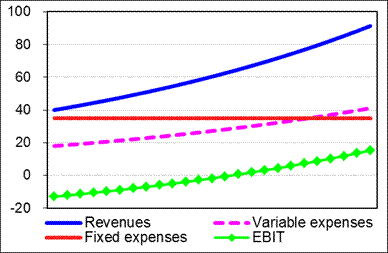Unlike other methods of valuation of loss-making companies, which rely primarily on use of comparable valuation ratios, and, therefore, provide only relative valuation, the Chepakovich Valuation Model estimates intrinsic (i.e. fundamental) value.

The calculation algorithm of splitting historical expenses as they reported in the income statement into fixed and variable expenses is an integral part of the Chepakovich Valuation Model. It is based on the reasoning outlined below.

First, let's write an equation for expenses growth rate: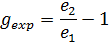(1)

where e1 and e2 are total expenses in two consecutive periods (Year 1 and Year 2, respectively).

Taking into account that total expenses could be split into fixed and variable, and assuming that fixed expenses do not change from year to year while variable expenses change at the rate of revenue growth, Equation (1) could be rewritten as follows: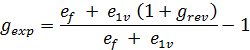(2)

where ef and e1v are, respectively, fixed and variable expenses in Year 1 and grev is revenue growth rate.

Equation (2) can be rewritten in the following way: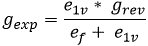(3)

Equation (3) can be rearranged: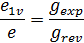(4)

where e is the total expenses (a sum of variable and fixed expenses) in Year 1.

From Equation (4) follows, that the ratio of variable expenses to total expenses, or the variable cost factor kv, as it is denoted below, is equal to the ratio of expenses growth rate to revenue growth rate: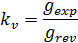(5)

both of which are quite easy to calculate using historical financial statements (revenues and expenses should be adjusted first for effects of acquisitions and divestments).

Other distinguishing and original features of the Chepakovich Valuation Model are:

• Variable discount rate (depends on time in the future from which cash flow is discounted to the present) to reflect investor's required rate of return (it is constant for a particular investor) and risk of investment (it is a function of time and riskiness of investment). The base for setting the discount rate is the so-called risk-free rate, i.e. the yield on a corresponding zero-coupon Treasury bond. The riskiness of investment is quantified through use of a risk-rating algorithm, which takes as inputs company-specific (‘funds from operations-to-debt’ and ‘net debt-to-capital’ ratios), which after incorporation of general macroeconomic (inflation, risk-free interest rate and equity risk premium) parameters transforms into the base discount rate (BDR). The BDR set for cash to be received in one year from now. To account for higher risk associated with further-in-future cash flows, a multiplier is applied in each consecutive year to the discount rate.
• Company's investments in means of production, or production assets as they are also referred to at this site (it is the sum of tangible and intangible assets needed for a company to produce a certain amount of output) is set to be a function of the revenue growth (there should be enough production capacity to provide increase in production/revenue). Surprisingly many discounted cash flow (DCF) models used today do not account for additional production capacity need when revenues grow.
• Long-term convergence of company's revenue growth rate to that of GDP. This follows from the fact that combined revenue growth rate of all companies in an economy is equal to the GDP growth rate and from an assumption that over- or underperformance (compared to the GDP) by individual companies will be eliminated in the long run (which is usually the case for the vast majority of companies - so vast, indeed, that the incompliant others could be treated as a statistical error). Valuation is conducted on the premise that change in the company's revenue is attributable only to the company's organic growth rate. This means that historical revenue growth rates are adjusted for effects of acquisitions/divestitures.
• Actual cost of stock-based compensation of company's employees that does not show in the company's income statement is subtracted from the cash flow. It is determined as the difference between the amount the company could have received by selling the shares at market prices and the amount it received from selling the shares to employees (the actual process of stock-based compensation could be much more complicated than the one described here, but its economic consequences are still the same). The actual cost of stock-based compensation (ACSBC) is calculated in the model as follows:
•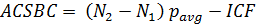(6)

where N1 and N2 are the number of shares outstanding at consecutive reporting dates 1 and 2, respectively, pavg is the average price of the company's stock during the period between the two reporting dates, and ICF is the net cash flow from issuance of shares as reported in the company's cash flow statement. This method provides an approximation of the ACSBC. The exact number could be calculated from data provided in the statement of shareholders' equity and notes to financial statements.

• It is assumed that, subject to availability of the necessary free cash flow, the company's capital structure (debt-to-equity ratio) will converge to optimal. This would also have an effect on the risk rating of the company and the discount rate. The optimal capital structure is defined as the one at which the sum of the cost of debt (company's interest payments) and its cost of equity (yield on an alternative investment with the same risk - it is a function of the company's financial leverage) after an adjustment for risk caused by business cycles is at its minimum. If the company is underleveraged, the model assumes that it will raise debt and payout an extraordinary dividend if this extra cash could not be efficiently deployed (i.e. if it will exceed what is required to achieve organic revenue growth rate targets). If, on the other hand, the company is overleveraged, then revenue growth is curtailed to reduce the level of debt.

#### Application of the Model

To calculate intrinsic value of a company's common stock, the Chepakovich valuation model needs only a limited number of input parameters (see the full list below). Most of them are derived directly from the company's financial statements, some are reflective of the current macroeconomic/market situation and are used for determining an appropriate initial discount rate, while the rest, though labeled below as industry-specific, allow for a certain degree of subjectivity.

MODEL'S INPUT PARAMETERS

Company-specific:

• Revenue
• Initial revenue growth rate
• Gross margin (excluding depreciation)
• Fixed operating expenses
• Interest rate on debt
• Corporate tax rate
• Cost of stock options / Revenue
• Dividend payout ratio
• Revenue / Production assets
• Revenue / Working capital
• Cash & short-term investments
• Goodwill
• Gross debt
• Equity
• Funds from operations
• Number of shares outstanding

Macroeconomic/market data:

• Risk-free rate
• Inflation rate